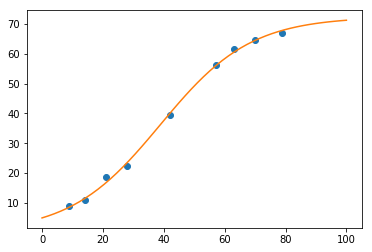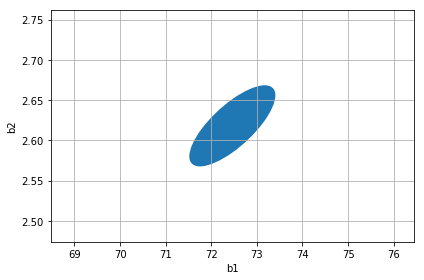# MultiNorm¶

This is a tutorial notebook for the MultiNorm class from the multinorm Python package (Github).

• A quick tutorial introduction (~ 10 min read)
• Explain and illustrate some of the theory / statistical aspects
• Hands-on introduction how to work with MultiNorm class.

Suggestions:

• Read this tutorial and judge if multinorm is useful for you.
• If yes, install it with pip install multinorm, download this notebook, and execute / play with these examples on your machine.

We will use pandas, so after reading this you will be a data scientist! In the future we will probably change the example model to a deep neural network and use tensorflow, because likelihood fitting is so 1990s.

## Introduction¶

We will be using scipy.optimize.curve_fit to fit a model to data and obtain an example covariance matrix. Note that most analysis problems are not a simple least squares curve fit, but involve custom data and likelihood. Information how to obtain parameter error and covariance estimates with other popular Python packages is described in the multinorm documentation.

## Imports¶

Let's start by importing everything we'll use in this notebook.

In :
import matplotlib.pyplot as plt
import numpy as np
import pandas as pd
import scipy.optimize
from multinorm import MultiNorm


## Example Setup¶

As mentioned in the introduction, the kind of analysis we are considering here is that there is a model with parameters, as well as some data and a likelihood function.

We will use the Rat42 dataset from the NIST Standard Reference Database. To quote from here:

This model and data are an example of fitting sigmoidal growth curves taken from Ratkowsky (1983). The response variable is pasture yield, and the predictor variable is growing time.

Did I hear you say

Why? pasture yield? WTF?

Well, the data is small (9 observations x, y) and , and the model is simple (one line in Python) and has 3 parameters, which is nice to illustrate properties of the correlation matrix and multivariate normal distribution. And the fit is using a least square likelihood, which is built-in in scipy and available for pretty much any fitting package, or can be implemented also with one line in Python.

In :
data = pd.DataFrame({
'x': [9, 14, 21, 28, 42, 57, 63, 70, 79],
'y': [8.93, 10.80, 18.59, 22.33, 39.35, 56.11, 61.73, 64.62, 67.08],
})
data.T

Out:
0 1 2 3 4 5 6 7 8
x 9.00 14.0 21.00 28.00 42.00 57.00 63.00 70.00 79.00
y 8.93 10.8 18.59 22.33 39.35 56.11 61.73 64.62 67.08

And having a DOI (http://dx.doi.org/10.18434/T43G6C) and expected reference results for the analysis is also nice. This is the result we should find:

In :
expected = pd.DataFrame.from_records(
[
("b1", 7.2462237576e01, 1.7340283401e00),
("b2", 2.6180768402e00, 8.8295217536e-02),
("b3", 6.7359200066e-02, 3.4465663377e-03),
],
columns=["par", "value", "error"],
index='par',
)
expected

Out:
value error
par
b1 72.462238 1.734028
b2 2.618077 0.088295
b3 0.067359 0.003447

### Model¶

Our model is just a simple line.

In :
def model(x, b1, b2, b3):
return b1 / (1 + np.exp(b2 - b3 *x))


### Fit¶

If we fit the model to the data, we obtain a parameter estimate (popt, the "optimial" or "best" parameter values) and a covariance matrix (pcov).

In :
p0 = (100, 1, 0.1)
popt, pcov = scipy.optimize.curve_fit(
f=model,
xdata=data["x"],
ydata=data["y"],
p0=p0,
)


Let's make a plot to see the best-fit model and the data:

In :
plt.plot(data['x'], data["y"], 'o')
x = np.linspace(0, 100)
plt.plot(x, model(x, *popt));Finally, this is where MultiNorm comes in!

## MultiNorm¶

In the last section we fit our model y = m x + b with two parameters (m, b) to some data, and optained the best-fit parameter vector popt and a covariance matrix pcov. Using this information, we can create a MultiNorm object.

### Create¶

In :
from multinorm import MultiNorm

In :
mn = MultiNorm(mean=popt, cov=pcov, names=["b1", "b2", "b3"])

In :
mn

Out:
MultiNorm with n=3 parameters:
mean       error
name
b1    72.462232  1.734039
b2     2.618077  0.088295
b3     0.067359  0.003447

MultiNorm is very simple, it just stores the mean vector and cov matrix and exposes them as pandas Series and DataFrame objects, with name as index.

Of course the mean and cov are still available as Numpy arrays, using the .values property of the pandas objects.

In :
mn.names

Out:
['b1', 'b2', 'b3']
In :
mn.mean

Out:
name
b1    72.462232
b2     2.618077
b3     0.067359
Name: mean, dtype: float64
In :
mn.cov

Out:
name b1 b2 b3
name
b1 3.006892 -0.069747 -0.005014
b2 -0.069747 0.007796 0.000250
b3 -0.005014 0.000250 0.000012

### Errors¶

In the printout of mn above, we saw that in addition to the names, mean and cov we passed in, another property error exists.

This is the vector of "1 sigma" parameter error estimates:

In :
mn.error

Out:
name
b1    1.734039
b2    0.088295
b3    0.003447
Name: error, dtype: float64

Usually you would state your measurement giving the estimated value and error for each parameter:

• b1 = 72.46 +/- 1.73
• b2 = 2.618 +/- 0.088
• b3 = 0.0673 +/- 0.0034

The relationship between the covariance matrix and the errors is very simple: the diagonal entries of the covariance matrix contains the errors squared, meaning that you can compute the errors as the square root of the diagonal entries:

In :
np.sqrt(pcov.diagonal())

Out:
array([1.73403926, 0.08829495, 0.00344656])

### Parameters¶

Possibly the property you'll use most is parameters, which is a pandas.DataFrame with name as index, and columns that contain mean and error for all parameters. It has a nice table printout, and you can use the common pandas methods to select rows and columns and entries.

In :
mn.parameters

Out:
mean error
name
b1 72.462232 1.734039
b2 2.618077 0.088295
b3 0.067359 0.003447
In :
pars = mn.parameters
# Access parameter info as Series by index or name
pars.iloc
pars.loc["b1"]
# All the power of pandas at your fingertip
# Just as an example:
pars["mean"].to_frame().T

Out:
name b1 b2 b3
mean 72.462232 2.618077 0.067359

### Correlations¶

tbd

In :
mn.correlation

Out:
name b1 b2 b3
name
b1 1.000000 -0.455547 -0.838906
b2 -0.455547 1.000000 0.821333
b3 -0.838906 0.821333 1.000000
In :
mn.correlation.loc["b1", "b3"]

Out:
-0.8389061143534849
In :
mn.correlation["b3"].to_frame()

Out:
b3
name
b1 -0.838906
b2 0.821333
b3 1.000000

### Plot¶

TODO: plotting ellipses with MPL is horrible. Add a plot helper method!

In :
import matplotlib.pyplot as plt
fig, ax = plt.subplots()
ellipse = mn.fix("b3").to_matplotlib_ellipse()
ax.set_xlabel("b1")
ax.set_ylabel("b2")
# ax.set_xlim(7, 13)
# ax.set_ylim(17, 23)
ax.grid(True)

plt.plot(mn.mean["b1"], mn.mean["b2"])
plt.tight_layout()

plt.show()## Summary¶

Let us recap the most important points concerning multivariante normal distributions and the MultiNorm class.

### Theory¶

• TODO: MVN are ...

### Code¶

• TODO: the MultiNorm class ...

## Exercises¶

• TODO
In [ ]: